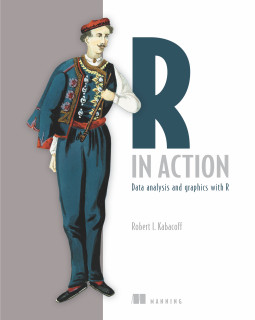# R语言在数据处理上的禀赋——par参数详解(一)

## 颜色相关

bg  # 背景色
fg  # 前景色
col # 颜色


bg和fg和含义一般编程语言中颜色的含义相同，下面举例说明一下：

opar<-par(no.readonly = TRUE)
x <- seq(1,10,length.out = 100) -5
y <- c(log(x[x>0]),log(abs(x[x<=0]))) #控制x大于0,看起来像乳房，怪数学

par(fg="red") #也可以写成类似 #ffffff形式
par(bg="black")
plot(x,y,type="l")
title(main="Black bg And Red fg",
col.main="yellow", #col的用法，下面介绍
sub="By program-dog.blogspot.com", #版权信息
col.sub="white"
)
par(opar)x <- seq(1,10,length.out = 100) -6
y <- x^2+10
z <- x^2+10
plot(x,y,type="l",col="red",ylim=c(5,15))
lines(x,z,type="l",col="green")
title(main="col的用法",
sub="By program-dog.blogspot.com" #版权信息
)col有一些参数是预设的，如下：

col.axis #坐标轴
col.lab  #标签
col.main #题目
col.sub  #副题目


## 字体相关

family #全局字体，特指字体的类型，如宋体还是楷体
font   #字体，特指字体的形态，如斜体还是粗体


opar<-par(no.readonly = TRUE)
par(mfrow=c(2,1))    #设置布局
x<- -10:10
y<- -(x^2)

# mono字体
par(family="mono")
plot(x,y,type='l')
title(main="family mono style",
sub="By program-dog.blogspot.com" #版权信息
)

# 默认字体
par(family="")
plot(x,y,type='l')
title(main="family default style",
sub="By program-dog.blogspot.com" #版权信息
)

par(opar)font.axis #坐标轴
font.lab  #标签
font.main #题目
font.sub #副题目


opar<-par(no.readonly = TRUE)
x<- seq(-10,10,length.out = 100)
y<- sin(x)
par(font.axis=1) # 1 普通文本
par(font.lab=2) # 2 粗体
par(font.main=3) # 3 斜体
par(font.sub=4) # 4 粗斜体
plot(x,y,type='l')
title(main="font style",
sub="By program-dog.blogspot.com" #版权信息
)
par(opar)font是用数字来指明的，再次提醒一下，这里的font代表的可不是特殊的字体哦。

## 字体大小相关

cex
ps #专门指字体


opar<-par(no.readonly = TRUE)
x<- seq(-10,10,length.out = 100)
y<- log(x^2)
par(mfrow=c(3,1))    #设置布局

par(ps=10) # 10
plot(x,y,type='l')
title(main="font size:ps=10",
sub="By program-dog.blogspot.com" #版权信息
)

par(ps=15) # 15
plot(x,y,type='l')
title(main="font size:ps=15",
sub="By program-dog.blogspot.com" #版权信息
)

par(ps=20) # 20
plot(x,y,type='l')
title(main="font size:ps=20",
sub="By program-dog.blogspot.com" #版权信息
)
par(opar)cex的用法也同于上面提到的col和font，几个预设的参数：

cex.axis #坐标轴
cex.lab  #标签
cex.main #题目
cex.sun  #副题目


opar<-par(no.readonly = TRUE)
x<- seq(-10,10,length.out = 100)
y<- sin(log(x^2))
par(mfrow=c(2,1))    #设置布局

par(cex.main=1)
plot(x,y,type='l')
title(main="font size:cex.main=1",
sub="By program-dog.blogspot.com" #版权信息
)

par(cex.main=1.5)
plot(x,y,type='l')
title(main="font size:cex.main=1.5",
sub="By program-dog.blogspot.com" #版权信息
)

par(opar)## 线条相关

lty #line style



x<- seq(-10,10,length.out = 100)
y<- sin(log(x^2))

par(lty=1) #lty 1
plot(x,-y,type='l',col="red",ylim=c(-3,3))
par(lty=2) #lty 2
lines(x,y,type='l',col="blue")
title(main="lty",
sub="By program-dog.blogspot.com" #版权信息
)
par(opar)lty也是用数字来表示不一样的线条风格，下面是在《R In Action》上弄下来的一张图：R In Action## 符号相关

opar<-par(no.readonly = TRUE)
x<- seq(-10,10,length.out = 20)
y1<- 0.1*x^2
y2<- 0.2*x^2
y3<- 0.4*x^2
y4<- 0.8*x^2
y5<- 1.6*x^2
y6<- 3.2*x^2

par(pch=1) #pch 1
plot(x,y1,type='b',col="red",xlim=c(0,5))
par(pch=2) #pch 2
lines(x,y2,type='b',col="blue")
par(pch=3) #pch 3
lines(x,y3,type='b',col="green")
par(pch=4) #pch 4
lines(x,y4,type='b',col="red")
par(pch=5) #pch 5
lines(x,y5,type='b',col="blue")
par(pch=6) #pch 6
lines(x,y6,type='b',col="green")

title(main="pch",
sub="By program-dog.blogspot.com" #版权信息
)
par(opar)pch的线条种类如下,图同样摘自《R In Action》## 线条和符号大小相关

opar<-par(no.readonly = TRUE)
x <- seq(1,10,length.out = 20)
y <- 1/x

par(mfrow=c(2,1))
plot(x,y,type="b",pch=2,cex=2,lty=3,lwd=2)
title(main="lwd =2  and cex = 2",
sub="By program-dog.blogspot.com" ,#版权信息
cex.sub=0.5
)
plot(x,y,type="b",pch=2,cex=1,lty=3,lwd=1)
title(main="lwd = 1 and cex =1",
sub="By program-dog.blogspot.com" ,#版权信息
cex.sub=0.5
)

par(opar)cex上面其实已经介绍过了，不过上面cex用在了par里面，相当于全局变量，会把所有的线条和符号（包括坐标轴，标题等）改变，而这里只是在plot的特定位置做了修饰，改变了局部。

## 结束

posted @ 2016-05-23 20:01  编程狗的博客  阅读(17199)  评论(0编辑  收藏